#### 期刊菜单

High School Mathematics Teaching Design Based on Problem Solving—Taking the Standard Equation of a Parabola as an Example
DOI: 10.12677/CES.2023.111023, PDF, HTML, XML, 下载: 22  浏览: 63  科研立项经费支持

Abstract: Problem-solving is the focus of today’s mathematics education circle, is a breakthrough of mathematics reform, is the new trend of mathematics education development. Problem solving ability is the ability of students to put forward and understand problems from the perspective of mathematics, and to comprehensively apply the knowledge and skills they have learned to solve problems. The cultivation of problem solving ability runs through the whole mathematics classroom teaching. Taking the teaching design of “the standard equation of parabola” as an example, this paper expounds on how to cultivate students’ problem-solving ability in the new teaching from three aspects, such as teaching idea, case description and teaching reflection.

1. 引言

2. 高中数学新授课问题解决教学思路

2.1. 创设情境，激发学生学习兴趣

2.2. 师生互动，激活课堂激活问题

2.3. 合作学习，交流分析解决问题

3. 教学设计

3.1. 创设情境，问题提出

3.2. 动手实践，探究新知Figure 1. Light reflection diagram of inner wall section of flashlight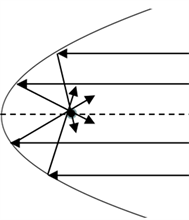Figure 2. Light reflection diagram of the cross section of the inner wall of a flashlight illuminated by parallel rays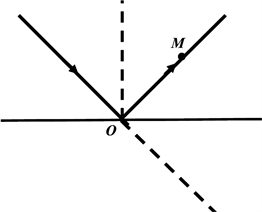Figure 3. Specular reflection of lightFigure 4. Specular reflection of light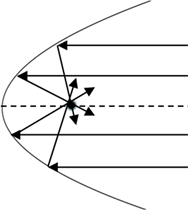Figure 5. Light reflection diagram of a cross-section surface of a parabolic inner wall illuminated by parallel rays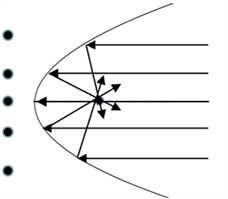Figure 6. The image of a point on a parabola with respect to the focus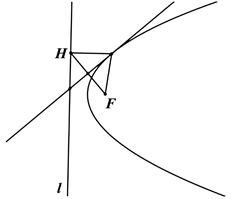Figure 7. A geometric drawing board makes a parabola

3.3. 合作探究，建构概念Table 1. System resulting data of standard experimentTable 2. Four standard equations for a parabola

3.4. 典例分析，巩固提高

(2) 二次函数 $y=a{x}^{2}\left(a\ne 0\right)$ 的图象是抛物线吗？求它的焦点坐标与准线方程。

4. 教学反思

4.1. 问题是数学的心脏，也是问题解决教学的切入点

4.2. 合作学习促进学生在问题解决过程中的主动参与

  易颖, 吴晓勤. 基于问题解决的初中数学教学设计——以“解直角三角形的应用”教学为例[J]. 亚太教育, 2019(11): 96-97.  王红革. 浅谈高中数学教学中学生问题解决能力的培养[J]. 天津市教科院学报, 2010(3): 94-95.  张晶, 夏小刚. 数学问题情境化设计中的认知偏差及任务靶向[J]. 数学教育学报, 2022, 31(6): 75-79.  姬梁飞. 数学问题解决素养的生成与培育[J]. 教学与管理, 2021, 830(1): 40-42.  戚洪祥. 数学问题解决: 演变、内涵及实践路径[J]. 上海教育科研, 2020, 402(11): 88-92.